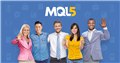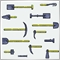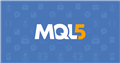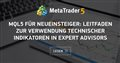# get SimpleMAOnBuffer value12

Hi, i want to get the value of the "SimpleMAOnBuffer(rates_total,prev_calculated,0,InpSignalMA,ExtMacdBuffer,ExtSignalBuffer);" but u only get 105577.0 and i want the value from the

curve, can someone explain me how ?

```//+------------------------------------------------------------------+
//|                                            MACD Histogram MC.mq5 |
//|                                                                  |
//|                                                        Based on: |
//|                                                         MACD.mq5 |
//|                        Copyright 2009, MetaQuotes Software Corp. |
//|                                              http://www.mql5.com |
//+------------------------------------------------------------------+
#property description "Moving Average Convergence/Divergence, Histogram, Multi-color"
/*--------------------------------------------------------------------
2010 10 20: v04   Fixed bug with the colors of the histogram

2010 09 26: v03   Added MODE_SMMA and MODE_LWMA as MA methods for Signal line
Made ENUM_APPLIED_PRICE to the end of the input parameter list
----------------------------------------------------------------------*/
#include <MovingAverages.mqh>
#property indicator_separate_window
#property indicator_buffers 6
#property indicator_plots   3
//--- indicator plots
#property indicator_type1   DRAW_LINE
#property indicator_type2   DRAW_LINE
#property indicator_type3   DRAW_COLOR_HISTOGRAM
#property indicator_color1  Blue
#property indicator_color2  Red
#property indicator_color3  Green,Red,Blue
#property indicator_width1  1
#property indicator_width2  1
#property indicator_width3  1
#property indicator_label1  "MACD"
#property indicator_label2  "Signal"
#property indicator_label3  "Histogram"
//--- enum variables
enum colorswitch                                         // use single or multi-color display of Histogram
{
MultiColor=0,
SingleColor=1
};

//--- input parameters
input int                  InpFastEMA=12;                // Fast EMA period
input int                  InpSlowEMA=26;                // Slow EMA period
input int                  InpSignalMA=9;                // Signal MA period
input ENUM_MA_METHOD       InpAppliedSignalMA=MODE_SMA;  // Applied MA method for signal line
input colorswitch          InpUseMultiColor=MultiColor;  // Use multi-color or single-color histogram
input ENUM_APPLIED_PRICE   InpAppliedPrice=PRICE_CLOSE;  // Applied price
//--- indicator buffers
double                     ExtMacdBuffer[];
double                     ExtSignalBuffer[];
double                     ExtHistogramBuffer[];
double                     ExtHistogramColorBuffer[];
double                     ExtFastMaBuffer[];
double                     ExtSlowMaBuffer[];
//--- global variables
int                        weightsum_global;             // used for calculation of LWMA
//--- indicator handles
int                        ExtFastMaHandle;
int                        ExtSlowMaHandle;
//--- turn on/off error messages
bool                       ShowErrorMessages=true;       // turn on/off error messages for debugging

//+------------------------------------------------------------------+
//| Custom indicator initialization function                         |
//+------------------------------------------------------------------+
void OnInit()
{
//--- indicator buffers mapping
SetIndexBuffer(0,ExtMacdBuffer,INDICATOR_DATA);
SetIndexBuffer(1,ExtSignalBuffer,INDICATOR_DATA);
SetIndexBuffer(2,ExtHistogramBuffer,INDICATOR_DATA);
SetIndexBuffer(3,ExtHistogramColorBuffer,INDICATOR_COLOR_INDEX);
SetIndexBuffer(4,ExtFastMaBuffer,INDICATOR_CALCULATIONS);
SetIndexBuffer(5,ExtSlowMaBuffer,INDICATOR_CALCULATIONS);

//--- set buffers as series, most recent entry at index 
ArraySetAsSeries(ExtMacdBuffer,true);
ArraySetAsSeries(ExtSignalBuffer,true);
ArraySetAsSeries(ExtHistogramBuffer,true);
ArraySetAsSeries(ExtHistogramColorBuffer,true);
ArraySetAsSeries(ExtFastMaBuffer,true);
ArraySetAsSeries(ExtSlowMaBuffer,true);

//--- sets first bar from what index will be drawn
PlotIndexSetInteger(0,PLOT_DRAW_BEGIN,InpSlowEMA-1);
PlotIndexSetInteger(1,PLOT_DRAW_BEGIN,InpSlowEMA+InpSignalMA-1);
PlotIndexSetInteger(2,PLOT_DRAW_BEGIN,InpSlowEMA+InpSignalMA-1);

//--- name for indicator
IndicatorSetString(INDICATOR_SHORTNAME,"MACDCustom("+string(InpFastEMA)+","+string(InpSlowEMA)+","+string(InpSignalMA)+")");

//--- get MA handles
ExtFastMaHandle=iMA(NULL,0,InpFastEMA,0,MODE_EMA,InpAppliedPrice);
ExtSlowMaHandle=iMA(NULL,0,InpSlowEMA,0,MODE_EMA,InpAppliedPrice);

//--- initialize global variable used for LWMA
weightsum_global=0;

//--- initialization done
}
//+------------------------------------------------------------------+
//| Moving Averages Convergence/Divergence                           |
//+------------------------------------------------------------------+
int OnCalculate(const int rates_total,
const int prev_calculated,
const datetime &Time[],
const double &Open[],
const double &High[],
const double &Low[],
const double &Close[],
const long &TickVolume[],
const long &Volume[],
{
//--- check for data
if(rates_total<InpSignalMA)
return(0);

//--- not all data may be calculated
int calculated;

calculated=BarsCalculated(ExtFastMaHandle);
if(calculated<rates_total)
{
if(ShowErrorMessages) Print("Not all data of ExtFastMaHandle has been calculated (",calculated,"bars ). Error",GetLastError());
return(0);
}

calculated=BarsCalculated(ExtSlowMaHandle);
if(calculated<rates_total)
{
if(ShowErrorMessages) Print("Not all data of ExtSlowMaHandle has been calculated (",calculated,"bars ). Error",GetLastError());
return(0);
}

//--- calculate how many bars need to be recalculated
int to_copy;
if(prev_calculated>rates_total || prev_calculated<0)
to_copy=rates_total;
else
{
to_copy=rates_total-prev_calculated;
if(prev_calculated>0)
to_copy++;
}

//--- get fast MA buffer values
if(CopyBuffer(ExtFastMaHandle,0,0,to_copy,ExtFastMaBuffer)<=0)
{
if(ShowErrorMessages) Print("Getting fast EMA failed! Error",GetLastError());
return(0);
}

//--- get slow MA buffer values
if(CopyBuffer(ExtSlowMaHandle,0,0,to_copy,ExtSlowMaBuffer)<=0)
{
if(ShowErrorMessages) Print("Getting slow SMA failed! Error",GetLastError());
return(0);
}

//--- set limit for which bars need to be (re)calculated
int limit;
if(prev_calculated==0 || prev_calculated<0 || prev_calculated>rates_total)
limit=rates_total-1;
else
limit=rates_total-prev_calculated;
//--- older bars () are needed to set the color of the current bar
if(limit>rates_total-1-1) limit=rates_total-1-1;

//--- calculate MACD buffer
for(int i=limit;i>=0;i--)
ExtMacdBuffer[i]=ExtFastMaBuffer[i]-ExtSlowMaBuffer[i];

//--- calculate Signal buffer
if(InpAppliedSignalMA==MODE_SMA)
SimpleMAOnBuffer(rates_total,prev_calculated,0,InpSignalMA,ExtMacdBuffer,ExtSignalBuffer);
if(InpAppliedSignalMA==MODE_EMA)
ExponentialMAOnBuffer(rates_total,prev_calculated,0,InpSignalMA,ExtMacdBuffer,ExtSignalBuffer);
if(InpAppliedSignalMA==MODE_SMMA)
SmoothedMAOnBuffer(rates_total,prev_calculated,0,InpSignalMA,ExtMacdBuffer,ExtSignalBuffer);
if(InpAppliedSignalMA==MODE_LWMA)
LinearWeightedMAOnBuffer(rates_total,prev_calculated,0,InpSignalMA,ExtMacdBuffer,ExtSignalBuffer,weightsum_global);

for(int i=limit;i>=0;i--)
{
//--- calculate Histogram buffer
ExtHistogramBuffer[i]=ExtMacdBuffer[i]-ExtSignalBuffer[i];
//--- set color Histogram
if(InpUseMultiColor==MultiColor)
{
if(ExtHistogramBuffer[i]>ExtHistogramBuffer[i+1])
ExtHistogramColorBuffer[i]=0;
if(ExtHistogramBuffer[i]<ExtHistogramBuffer[i+1])
ExtHistogramColorBuffer[i]=1;
if(ExtHistogramBuffer[i]==ExtHistogramBuffer[i+1])
ExtHistogramColorBuffer[i]=2;
}
if(InpUseMultiColor==SingleColor)
ExtHistogramColorBuffer[i]=0;
}

//--- return value of rates_total, will be used as prev_calculated in next call
return(rates_total);
}
//+------------------------------------------------------------------+```Открой новые возможности в MetaTrader 5 с сообществом и сервисами MQL5
• 2022.08.02
• www.mql5.com
MQL5: язык торговых стратегий для MetaTrader 5, позволяет писать собственные торговые роботы, технические индикаторы, скрипты и библиотеки функций1249

It looks quite okay but I think you are making it way too complicated by using so many buffers. Try building an Indicator with one or two buffers and when that work take it from there... then you would have less error sources.

Question: Does it compile without errors?
Why did you set the buffers as series?12

Tobias Johannes Zimmer #:
It looks quite okay but I think you are making it way too complicated by using so many buffers. Try building an Indicator with one or two buffers and when that work take it from there... then you would have less error sources.

Question: Does it compile without errors?
Why did you set the buffers as series?
I have no error when i compile and it's not my indicator, i take it from the community.
this is why it's difficult for me to modify this, and i just want to get the signal value with EMA smoothing.
Sorry if i don't speack properly but i'm not English.380

SuzuA #:
I have no error when i compile and it's not my indicator, i take it from the community.
this is why it's difficult for me to modify this, and i just want to get the signal value with EMA smoothing.
Sorry if i don't speack properly but i'm not English.

Have a look at the documentation, they have an example there too

https://www.mql5.com/en/docs/indicators/imaDocumentation on MQL5: Technical Indicators / iMA
• www.mql5.com
iMA - Technical Indicators - MQL5 Reference - Reference on algorithmic/automated trading language for MetaTrader 51249

I don't quite understand what your question is really about. MovingAverages.mqh is a file from the Include folder that is declared in the head of your code so its functions can be used.

```

#include <MovingAverages.mqh>
#property indicator_separate_window
#property indicator_buffers 6
..........
//--- calculate Signal buffer    if(InpAppliedSignalMA==MODE_SMA)       SimpleMAOnBuffer(rates_total,prev_calculated,0,InpSignalMA,ExtMacdBuffer,ExtSignalBuffer);    if(InpAppliedSignalMA==MODE_EMA)       ExponentialMAOnBuffer(rates_total,prev_calculated,0,InpSignalMA,ExtMacdBuffer,ExtSignalBuffer);    if(InpAppliedSignalMA==MODE_SMMA)       SmoothedMAOnBuffer(rates_total,prev_calculated,0,InpSignalMA,ExtMacdBuffer,ExtSignalBuffer);    if(InpAppliedSignalMA==MODE_LWMA)       LinearWeightedMAOnBuffer(rates_total,prev_calculated,0,InpSignalMA,ExtMacdBuffer,ExtSignalBuffer,weightsum_global);```

The other thing is how can indicators be used in other programs, Expert Advisors: